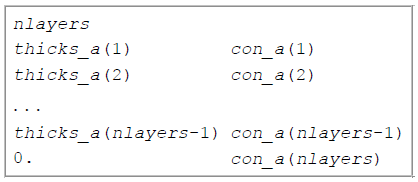# 5.3.1. 1D Mesh file¶

This is the file used to define a finite difference mesh for 1D modeling and inversion. In the file, the vertical column representing the earth is divided into layers. By convention, the z-axis is positive down.

An example of the file structure is as follows:• Number of segments used to define the vertical partitioning

• Column 1: From there, each line defines the layer thickness.

• Column 2 [OPTIONAL]: Model value corresponding to the $$i^{th}$$ layer